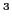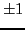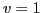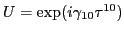Next: Constraints for the scalar-isovector Up: Continuity equation and local Previous: Constraints for the vector-isovector

# conclusions

In the present work, we have derived sets of constraints on the coupling constants of the NLO energy density functional that guarantee the validity of the continuity equation in the four spin-isospin channels. In the scalar-isoscalar channel, these constraints are identical to those induced by the standard local gauge invariance conditions. We extended this connection to vector and isovector channels, where the validity of the continuity equations is equivalent to the local gauge invariance with respect to spin and isospin rotations, respectively.

We note here that in our analysis we implicitly assumed that all densities that build the NLO energy density functional are nonzero. Of course, there can be many specific situations when some densities vanish, and thus the coupling constants related to them become unrestricted. This occurs, for instance, when densities are restricted by some symmetry conditions. Obviously, the methods developed in the present work can then be applied to derive new (weaker) sets of constraints that correspond to each one particular case. For example, when the proton-neutron symmetry is conserved, see, e.g., Refs. [16,18], thecomponents of all isovector densities () vanish, and the energy density is invariant with respect to a one-dimensional U(1) gauge rotation in the isospin space, that is, with respect to. Then, the continuity equations for neutrons and protons decouple from one another and become independently valid, provided the isoscalar and isovector coupling constants independently obey the standard gauge-invariance conditions.

This work was supported in part by the Academy of Finland and the University of Jyväskylä within the FIDIPRO programme, and by the Polish Ministry of Science and Higher Education.Next: Constraints for the scalar-isovector Up: Continuity equation and local Previous: Constraints for the vector-isovector
Jacek Dobaczewski 2011-11-11